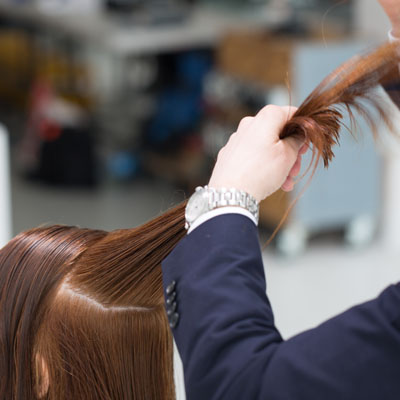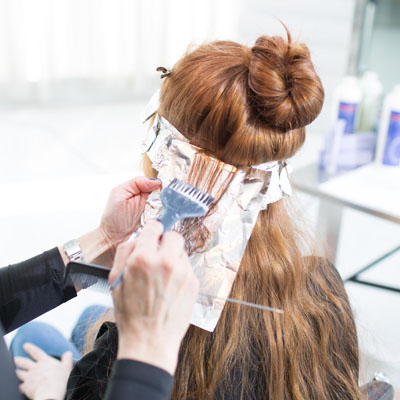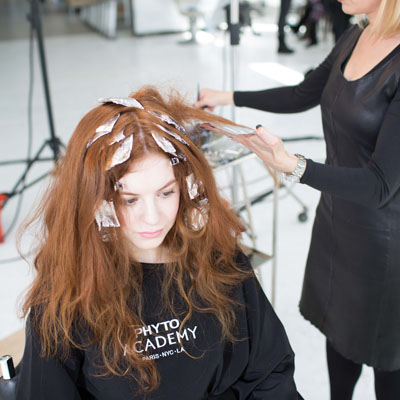August 30, 2016

# Curly Cut and Rich Red

VIEW ALL PHOTOS VIEW SLIDESHOW
GET THE HOW-TO• Formula A

PHYTO SUBTIL CRÈME ICE + 40-volume PHYTO SUBTIL Blond developing cream

• Formula B

SUBTIL CRÈME ICE + 30-volume SUBTIL Blond developing cream

• Formula C

SUBTIL CRÈME ICE + 20-volume SUBTIL Blond developing cream# Curly Cut and Rich Red

Rock ‘n’ roll is back, and styles from the era of big volume and curls are making a statement in a big way. For this look, the PHYTO team added depth, dimension and brightness to bring out the cool copper shade and enhance the curly, face-framing cut. Here’s how you can recreate the cut and color in your salon!

Colorist: Becky Janopoulos
Manufacturer: PHYTO, @phytousa

## COLOR FORMULAS• Formula A

PHYTO SUBTIL CRÈME ICE + 40-volume PHYTO SUBTIL Blond developing cream

• Formula B

SUBTIL CRÈME ICE + 30-volume SUBTIL Blond developing cream

• Formula C

SUBTIL CRÈME ICE + 20-volume SUBTIL Blond developing cream

## HOW-TO STEPS•• Formula A

PHYTO SUBTIL CRÈME ICE + 40-volume PHYTO SUBTIL Blond developing cream

• Formula B

SUBTIL CRÈME ICE + 30-volume SUBTIL Blond developing cream

• Formula C

SUBTIL CRÈME ICE + 20-volume SUBTIL Blond developing cream

1

Create a halo section that starts at the round of the forehead and runs across the crown.

•• Formula A

PHYTO SUBTIL CRÈME ICE + 40-volume PHYTO SUBTIL Blond developing cream

• Formula B

SUBTIL CRÈME ICE + 30-volume SUBTIL Blond developing cream

• Formula C

SUBTIL CRÈME ICE + 20-volume SUBTIL Blond developing cream

2

Comb everything down to 0 degrees, and cut the baseline in a square, turning the head to the side and connecting through.

•• Formula A

PHYTO SUBTIL CRÈME ICE + 40-volume PHYTO SUBTIL Blond developing cream

• Formula B

SUBTIL CRÈME ICE + 30-volume SUBTIL Blond developing cream

• Formula C

SUBTIL CRÈME ICE + 20-volume SUBTIL Blond developing cream

3

Taking vertical sections, lift the hair above 90 degrees and cut concave layers.

•• Formula A

PHYTO SUBTIL CRÈME ICE + 40-volume PHYTO SUBTIL Blond developing cream

• Formula B

SUBTIL CRÈME ICE + 30-volume SUBTIL Blond developing cream

• Formula C

SUBTIL CRÈME ICE + 20-volume SUBTIL Blond developing cream

4

Continue layering without overdirecting until you reach the ear, then bring the remaining hair on the sides back to the previous guide.

•• Formula A

PHYTO SUBTIL CRÈME ICE + 40-volume PHYTO SUBTIL Blond developing cream

• Formula B

SUBTIL CRÈME ICE + 30-volume SUBTIL Blond developing cream

• Formula C

SUBTIL CRÈME ICE + 20-volume SUBTIL Blond developing cream

5

Carve out a fringe that starts at the nose and connects to the outline.

•• Formula A

PHYTO SUBTIL CRÈME ICE + 40-volume PHYTO SUBTIL Blond developing cream

• Formula B

SUBTIL CRÈME ICE + 30-volume SUBTIL Blond developing cream

• Formula C

SUBTIL CRÈME ICE + 20-volume SUBTIL Blond developing cream

6

Lean the head forward, combing the hair toward the front, and continue refining the fringe.

•• Formula A

PHYTO SUBTIL CRÈME ICE + 40-volume PHYTO SUBTIL Blond developing cream

• Formula B

SUBTIL CRÈME ICE + 30-volume SUBTIL Blond developing cream

• Formula C

SUBTIL CRÈME ICE + 20-volume SUBTIL Blond developing cream

7

Start in the crown with horizontal sections, using the previously-cut hair as the guide. Lift hair up and back, then cut a flat layer. Continue using a stationary guide until you reach the hairline.

•• Formula A

PHYTO SUBTIL CRÈME ICE + 40-volume PHYTO SUBTIL Blond developing cream

• Formula B

SUBTIL CRÈME ICE + 30-volume SUBTIL Blond developing cream

• Formula C

SUBTIL CRÈME ICE + 20-volume SUBTIL Blond developing cream

8

At the high point of the crown using horizontal sections, lift the hair up and connect to the sides continuing to the hairline.

•• Formula A

PHYTO SUBTIL CRÈME ICE + 40-volume PHYTO SUBTIL Blond developing cream

• Formula B

SUBTIL CRÈME ICE + 30-volume SUBTIL Blond developing cream

• Formula C

SUBTIL CRÈME ICE + 20-volume SUBTIL Blond developing cream

9

Using vertical sections at the top of the head, connect the crown to the fringe.

•• Formula A

PHYTO SUBTIL CRÈME ICE + 40-volume PHYTO SUBTIL Blond developing cream

• Formula B

SUBTIL CRÈME ICE + 30-volume SUBTIL Blond developing cream

• Formula C

SUBTIL CRÈME ICE + 20-volume SUBTIL Blond developing cream

10

Pivot around the head to cross check.

•• Formula A

PHYTO SUBTIL CRÈME ICE + 40-volume PHYTO SUBTIL Blond developing cream

• Formula B

SUBTIL CRÈME ICE + 30-volume SUBTIL Blond developing cream

• Formula C

SUBTIL CRÈME ICE + 20-volume SUBTIL Blond developing cream

11

Taking vertical twisted sections, slide through to remove weight and release texture.

•• Formula A

PHYTO SUBTIL CRÈME ICE + 40-volume PHYTO SUBTIL Blond developing cream

• Formula B

SUBTIL CRÈME ICE + 30-volume SUBTIL Blond developing cream

• Formula C

SUBTIL CRÈME ICE + 20-volume SUBTIL Blond developing cream

12

Start with a halo section, leaving out 1.5 inches of hair, and apply Formula A with foils following a circular pattern and using a heavy weaving technique.

•• Formula A

PHYTO SUBTIL CRÈME ICE + 40-volume PHYTO SUBTIL Blond developing cream

• Formula B

SUBTIL CRÈME ICE + 30-volume SUBTIL Blond developing cream

• Formula C

SUBTIL CRÈME ICE + 20-volume SUBTIL Blond developing cream

13

Drop the next 1.5 inch section using a light weaving technique and following the same circular pattern.

•• Formula A

PHYTO SUBTIL CRÈME ICE + 40-volume PHYTO SUBTIL Blond developing cream

• Formula B

SUBTIL CRÈME ICE + 30-volume SUBTIL Blond developing cream

• Formula C

SUBTIL CRÈME ICE + 20-volume SUBTIL Blond developing cream

14

Continue to the next 1.5 inch circular section, and foil using Formula B in a heavy weaving technique, making sure to stay away from the part.

•• Formula A

PHYTO SUBTIL CRÈME ICE + 40-volume PHYTO SUBTIL Blond developing cream

• Formula B

SUBTIL CRÈME ICE + 30-volume SUBTIL Blond developing cream

• Formula C

SUBTIL CRÈME ICE + 20-volume SUBTIL Blond developing cream

15

With one long stroke, backcomb and apply color to the ends in foil.

•• Formula A

PHYTO SUBTIL CRÈME ICE + 40-volume PHYTO SUBTIL Blond developing cream

• Formula B

SUBTIL CRÈME ICE + 30-volume SUBTIL Blond developing cream

• Formula C

SUBTIL CRÈME ICE + 20-volume SUBTIL Blond developing cream

16

Drop another 1.5 inch section leaving a diamond shape at the top of the head that is subsectioned into quadrants, then apply a medium weaving technique to all remaining sections.

•• Formula A

PHYTO SUBTIL CRÈME ICE + 40-volume PHYTO SUBTIL Blond developing cream

• Formula B

SUBTIL CRÈME ICE + 30-volume SUBTIL Blond developing cream

• Formula C

SUBTIL CRÈME ICE + 20-volume SUBTIL Blond developing cream

17

Between the foils on the top, backcomb and apply Formula C to the ends.

•• Formula A

PHYTO SUBTIL CRÈME ICE + 40-volume PHYTO SUBTIL Blond developing cream

• Formula B

SUBTIL CRÈME ICE + 30-volume SUBTIL Blond developing cream

• Formula C

SUBTIL CRÈME ICE + 20-volume SUBTIL Blond developing cream

18

Finished Look